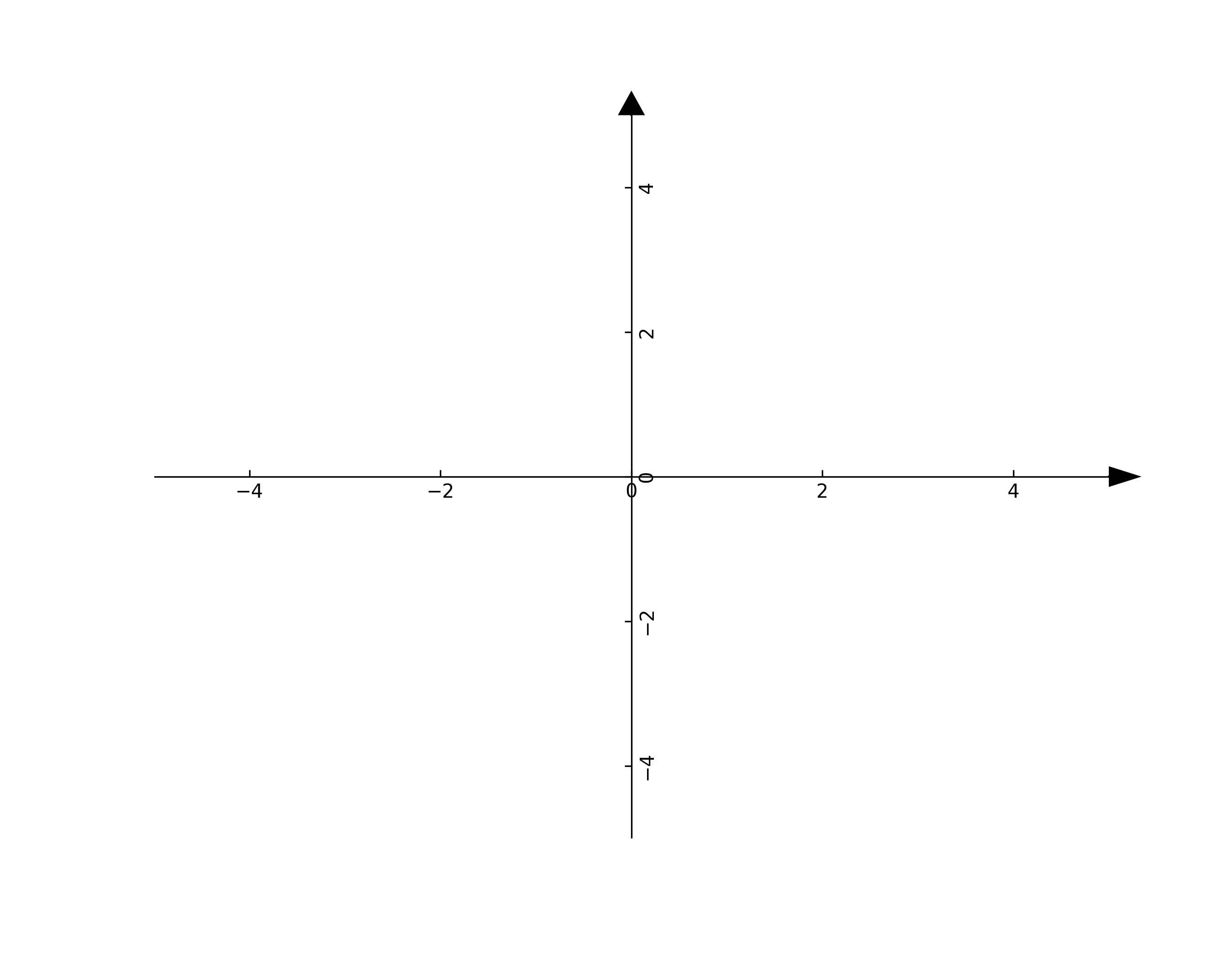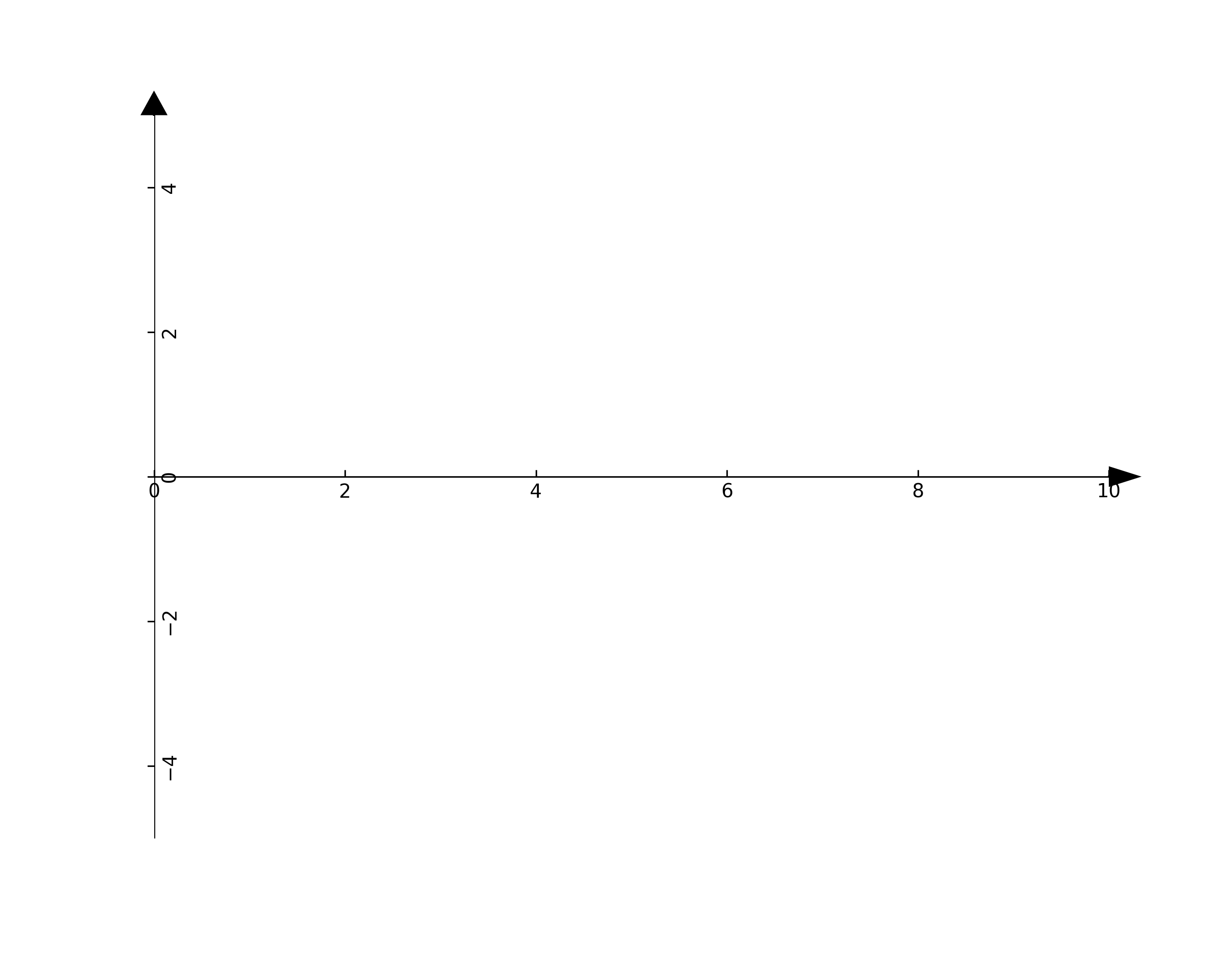# Drawing a Cartesian coordinate system in python

Again one of these things that maybe 100 other people will ever need to do in their lives. In case you are one of us though here is a short convenient Python class that will make your life easier 😀

EDIT: This script is now part of a package available through pip, so you can plot your coordinate system even faster! Details in this post.

```import numpy as np
import matplotlib.pyplot as plt
from mpl_toolkits.axisartist import SubplotZero

class Axes():

def __init__(self, xlim=(-5,5), ylim=(-5,5), figsize=(12,5)):
self.xlim = xlim
self.ylim = ylim
self.figsize  = figsize
self.__scale_arrows__()

def __drawArrow__(self, x, y, dx, dy, width, length):
plt.arrow(
x, y, dx, dy,
color       = 'k',
clip_on     = False,
)

def __scale_arrows__(self):
""" Make the arrows look good regardless of the axis limits """
xrange = self.xlim - self.xlim
yrange = self.ylim - self.ylim

def __drawAxis__(self):
"""
Draws the 2D cartesian axis
"""
# A subplot with two additional axis, "xzero" and "yzero"
# corresponding to the cartesian axis
ax = SubplotZero(self.fig, 1, 1, 1)

# make xzero axis (horizontal axis line through y=0) visible.
for axis in ["xzero","yzero"]:
ax.axis[axis].set_visible(True)
# make the other axis (left, bottom, top, right) invisible
for n in ["left", "right", "bottom", "top"]:
ax.axis[n].set_visible(False)

# Plot limits
plt.xlim(self.xlim)
plt.ylim(self.ylim)

# Draw the arrows
self.__drawArrow__(self.xlim, 0, 0.01, 0, 0.3, 0.2) # x-axis arrow
self.__drawArrow__(0, self.ylim, 0, 0.01, 0.2, 0.3) # y-axis arrow

def draw(self):
# First draw the axis
self.fig = plt.figure(figsize=self.figsize)
self.__drawAxis__()

#--------------------------------------------------------
# Main Code
#--------------------------------------------------------
axes = Axes(xlim=(-5,5), ylim=(-5,5), figsize=(9,7))
axes.draw()```

Which producesIt is also very easy to change the axis limits

```# Create the cartesian axis
axes = Axes(xlim=(0,10), ylim=(-5,5), figsize=(9,7))
axes.draw()```